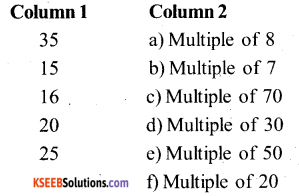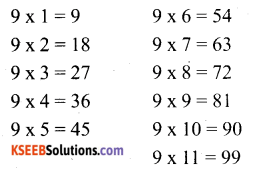# KSEEB Solutions for Class 6 Maths Chapter 3 Playing with Numbers Ex 3.1

Students can Download Chapter 3 Playing with Numbers Ex 3.1 Questions and Answers, Notes Pdf, KSEEB Solutions for Class 6 Maths helps you to revise the complete Karnataka State Board Syllabus and score more marks in your examinations.

## Karnataka State Syllabus Class 6 Maths Chapter 3 Playing with Numbers Ex 3.1

Question 1.
Write all the factors of the following numbers:
a) 24
b) 15
c) 21
d) 27
e) 12
f) 20
g) 18
h) 23
i) 36
Solution:
a) 24 :-
24 = 1 × 24, 24 = 2 × 12; 24 = 3 × 8
= 24 = 4 × 6 ; 24 = 6 x 4 ;
∴ Factors of 24 are 1, 2, 3, 4, 6, 8, 12 & 24

b) 15:-
15 = 1 × 15; 15 = 3 × 5; 15 = 5 × 3
∴ Factors of 15 are 1, 3, 5 and 15

c) 21:-
21 = 1 × 21 ; 21 = 3 × 7; 21 = 7 × 3
∴ Factors of 21 are 1, 3, 7 and 21d) 27:-
27 = 1 × 27; 27 = 3 × 9; 21 =9 × 3
∴ Factors of 27 are 1, 3, 9 and 27

e) 12:-
12 = 1 × 12; 12 = 2 × 6; 12 = 4 × 3
∴ Factors of 12 are 1, 2, 3, 4, 6 and 12

f) 20:-
20 = 1 × 20 ; 20 = 2 × 10; 20 = 4 × 5; 20 = 5 × 4 ; 20 = 10 × 2
∴ Factors of20 are 1, 2, 4, 5, 10 and 20

g) 18:-
18 = 1 ×18; 18 = 2 × 9; 18 = 3 × 6; 18 = 6 × 3
∴ Factors of 18 are 1, 2, 3, 6, 9 and 18

h) 23:-
23 = 1 × 23 ; 23 = 23 × 1
∴ Factors of 23 are 1 and 23

i) 36:-
36 = 1 × 36; 36 = 2 × 18; 36 = 3 × 12; 36 = 4 × 9; 36 = 6 × 6
∴ Factors of 36 are 1, 2, 3, 4, 6, 9, 12, 18 and 36.Question 2.
Write first five multiples of:
a) 5
b) 8
c) 9
Solution:
a) 5 = 5 × 1= 5; 5 × 2 = 10; 5 × 3 = 15; 5 × 4 = 20; 5 × 5 = 25. 5, 10, 15, 2, 25
b) 8 = 8 × 1 = 8; 8 × 2 = 16; 8 × 3 = 24; 8 × 4 = 32; 8 × 5 =40. 8,16,24,32,40
c) 9 = 9 × 1 = 9; 9 × 2 = 18; 9 × 3 = 27; 9 × 4 = 36; 9 × 5 = 45. 9,18,27,36,45

Question 3.
Match the items in column 1 with the items in column 2.Solution:
1 – b,
2 – d,
3 – a,
4 – f,
5 – e.Question 4.
Find all the multiples of 9 upto 100.
Solution:Therefore the multiples of 9 up to 100 are
9, 18, 27, 36, 45, 54, 63, 72, 81, 90 and 99.

error: Content is protected !!